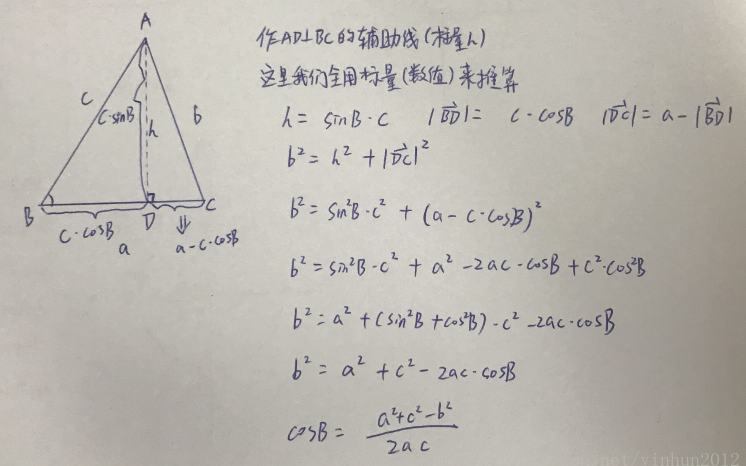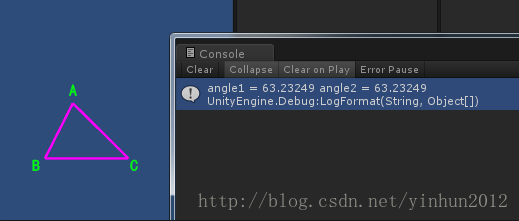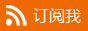#少有人走的路

## 三角函数：正弦余弦定理及应用

1.余弦定理公式1.正弦定理的推导，前面有余弦定理，那么肯定就有一个对应的正弦定理了。

ps：这里要了解一个外接圆的概念，就是三角形△ABC的三个顶点都在一个圆上，那么这个圆就是△ABC的外接圆，其实这个也好反向理解，比如我们先画一个圆，然后任意在圆上取三点，连接三点就是一个三角形，但是假如我们不知道三角形的外接圆，要怎么去绘画和证明呢，如下图：```using System.Collections;
using System.Collections.Generic;
using UnityEngine;

public class AngleFunc : MonoBehaviour {

public GameObject PointA;
public GameObject PointB;
public GameObject PointC;

void Start()
{
{  //构建一个三角形
GameObject[] gos = new GameObject;
gos = PointA;
gos = PointB;
gos = PointC;
for (int i = 0; i < gos.Length; i++)
{
line.positionCount = 2;
line.startWidth = 0.1f;
line.endWidth = 0.1f;
int index = i + 1;
if (index >= gos.Length)
index = 0;
line.SetPosition(0, gos[i].transform.position);
line.SetPosition(1, gos[index].transform.position);
}
}
//用余弦定理计算角度
Vector3 AB = PointB.transform.position - PointA.transform.position;
Vector3 BC = PointC.transform.position - PointB.transform.position;
Vector3 AC = PointC.transform.position - PointA.transform.position;
//计算∠A的夹角
float angle1 = Mathf.Acos((getVectorLengthPow2(AB) + getVectorLengthPow2(AC) - getVectorLengthPow2(BC)) / (2 * Mathf.Sqrt(getVectorLengthPow2(AB) * getVectorLengthPow2(AC)))) * Mathf.Rad2Deg;
//用自带的api计算角度
float angle2 = Vector3.Angle(AB, AC);
#if UNITY_EDITOR
Debug.LogFormat("angle1 = {0} angle2 = {1}", angle1, angle2);
#endif
}

private float getVectorLengthPow2(Vector3 vec)
{
return Mathf.Pow(vec.x, 2) + Mathf.Pow(vec.y, 2) + Mathf.Pow(vec.z, 2);
}

}```

---------------------

#转载请注明出处 www.skcircle.com 《少有人走的路》勇哥的工业自动化技术网站。如果需要本贴图片源码等资源，请向勇哥索取。

◎欢迎参与讨论，请在这里发表您的看法、交流您的观点。

«   2020年10月   »
1234
567891011
12131415161718
19202122232425
262728293031

查看权限

•• 扫描加本站机器视觉QQ群，验证答案为：halcon• 扫描加站长微信：• 扫描加站长QQ：• 扫描赞赏本站：